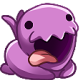Sijith -4 years ago 109
C Question

# Duplicate elements in array using Hash Table in C++

Hi Can some one give example of finding array duplicate using Hash table and function.

I am looking for some examples in c++
The codes I am getting is all on Javanefas

One solution is to put in a hash table the element of the array (as key) and their number of occurrence (as value). Then you copy the key of the hash table where the associated value is more than 1.

``````#include <string>
#include <vector>
#include <map>
#include <iostream>
#include <algorithm>

int main(int argc, char* argv[]) {
std::vector<int> vec{1,1,2,3,1,4,5};
std::map<int, int> m;
// We copy the element of the vector into the hash table
std::for_each(vec.begin(), vec.end(), [&m](auto & elt){ m[elt] += 1; });
std::vector<int> res;
// We select the key where the value is > 1
std::for_each(m.begin(), m.end(), [&res](auto & elt) { if(elt.second > 1) res.push_back(elt.first); });
}
``````
Recommended from our users: Dynamic Network Monitoring from WhatsUp Gold from IPSwitch. Free Download